Related Tags

arrays
python3

# What is bucket sort?

Imagine you come home after a long day and you are feeling hungry. You open the fridge to figure out what you want to eat for dinner, only to find out that all the food you have expires in the next 30 days! As a single-living millennial, this scenario is not strange. So, what do you do? Naturally, you want to prioritize the items whose expiration dates are closer and eat them all in order not to waste any. Sure, you can do this by hand, but as a computer scientist knows, you can’t just enter the number of days until expiration for every item to your computer and let a sorting algorithm figure it out.

Comparison Based Sorting Algorithms
As a computer scientist, you also know that there are numerous sorting algorithms that you can choose from. Insertion sort or selection sort are comparison-based algorithms, and they might be the first ones that one might think of because they are simple to understand and implement. Both insertion sort and selection sort have an average time complexity of O(n2), which quickly becomes an issue as n increases. To achieve better run-time, we can go for other comparison-based algorithms that have an average time complexity of O(nlogn) such as merge sort, quick sort, and heap sort. O(nlogn) also happens to be the lower bound for comparison-based sorting algorithms. In other words, you cannot get a better run time than O(nlogn) using a comparison-based sorting algorithm.

Scalability
What if you are known for your love of food, and you would like to be able to sort a lot of items? If you have the time, certainly all of these algorithms would work, but the question is can you do better? Can you sort faster than O(nlogn)? The answer is yes. “Wait,” you might say. Haven’t I just mentioned that you cannot get a better run time than O(nlogn) using a comparison-based sorting algorithm? The key detail here is the type of the sorting algorithm as there are sorting algorithms that are not comparison based. These algorithms can allow for sorting to take place in less than O(nlogn) time. One of these algorithms is bucket sort. If you are familiar with counting sort, you can think of bucket sort as a generalized case of counting sort.

Even though it is not necessary to understand this article, if you would like to read about counting sort you can visit this course.

How does bucket sort work?
Bucket sort is a sorting algorithm that divides the data points into their corresponding buckets, sorts each bucket individually, and recombines the sorted elements to yield an ordered dataset. Buckets are groups that the elements of the input are divided into. Depending on the input, they can be different numerical intervals (i.e., 0-5) or even letters of the alphabet (i.e., A-D). The number and range of the buckets depend on the size and content of the input elements.

The first step of bucket sort is to create the empty buckets. Afterwards, every element in the input is placed into their corresponding bucket. Then, all buckets are sorted separately. This can be done using any appropriate sorting algorithm, such as insertion sort and selection sort, or recursively calling bucket sort. Finally, the sorted buckets are gathered back together.

Pseudocode
1- Create n empty buckets
2- Place every element in their corresponding bucket
3- Sort individual buckets (ex: insertion sort)
4- Merge all the buckets


def bucket_sort (array_to_sort, n) :

#create list that will hold all the buckets (also lists)
bucket_list = []
bucket_number = n
while (bucket_number > 0):
new_bucket = []
bucket_list.append(new_bucket)
bucket_number = bucket_number - 1

maximum = max(array_to_sort)
minimum = min(array_to_sort)

#the numerical range that each bucket can hold
element_range = (maximum - minimum) // n+1

#place elements in their respective buckets
for index in range(len(array_to_sort)):
number = array_to_sort[index]
difference = number - minimum
bucket_number = (int) (difference // element_range)
bucket_list[bucket_number].append(number)

#sort all the buckets and merge them
sorted_list = []
for bucket in range(len(bucket_list)):
current = bucket_list[bucket]
current.sort()
if len(current) != 0:
sorted_list.extend(current)

return sorted_list

def main():
example_list = [3,1,5,2,1]
sorted_list = bucket_sort(example_list,5)
print(example_list)
print(sorted_list)

if __name__ == "__main__":
main()



Analysis of Time and Space Complexity
Creating m empty buckets takes O(m) time since creating one bucket takes O(1) time, and there are m buckets. Placing every element in their corresponding bucket will take O(n) time where n is the total number of elements to be sorted, assuming that we can perform multiplication and division in O(1) time. Merging m buckets at the end will take O(m) time. The most complex part of the run-time analysis is predicting the time it takes to sort the buckets. Using probability one can deduce that this process will take O(n2/k + n). Therefore, the overall runtime can be expressed as O(n2/k + n + k). Worst-case space complexity for bucket sort is O(nk).

Pros
In the average case, if n is similar to k, this expression will simplify to
O(n + k), so O(n). Therefore, with bucket sort, we can sort in linear time, which is faster than O(nlogn)!

Cons
If data is not uniformly distributed, worst-case run time is O(n2), which is slower compared to O(nlogn).

Bucket sort has worst case O(nk) space complexity, which is significantly greater than merge sort’s space complexity which is O(n).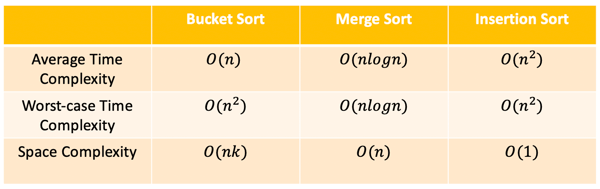Comparing Bucket Sort with comparison based sorting algorithms

Can we improve bucket sort?
There are numerous variants of bucket sort that aim to avoid the worst-case scenario. One of these variants is histogram sort. Histogram sort determines the number of elements that would fall into each bucket. With this information, boundaries of the buckets can be redefined, enabling the elements to be arranged into buckets in a more uniform way. This avoids the worst-case O(n2) time complexity.

Going back to our expiration date example…
Imagine you look at the items in your fridge and get the input array: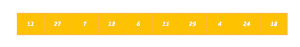You then distribute each element to their corresponding buckets: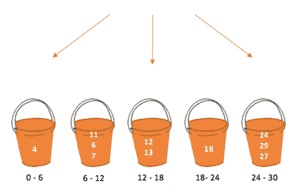Each bucket is sorted individually: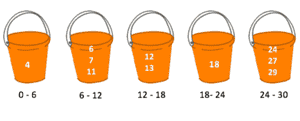The sorted elements are merged together to yield the sorted array!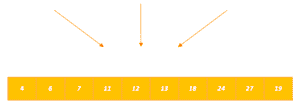RELATED TAGS

arrays
python3

CONTRIBUTOR